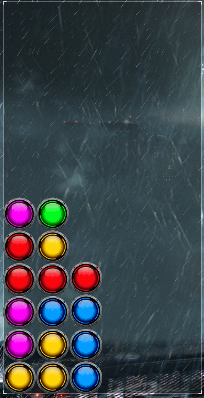# Score calculation bug

There is a case:It will be calculated as 480 points. But from formula:

score = (10 * B) * (CP + CB + GB)

How B calculated in this case? Seems it is
score = (10 * 4) * (8 + 4 + 0)

But question if for example blue will be 5 blocks and red 4. Which one calculator must choose?

Ah, ok. Found:

first step 802 = 160
second step: 40
8 = 320

total 480